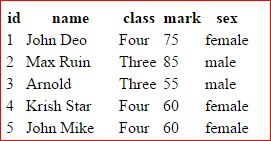# mysql_field_name Functions to get name of the fields of a table

By using the simple query 'SHOW COLUMNS FROM table' we can get the column names. This query will list out the column names. To display all the records of a table we will use this to show column names. Here is the sample code.
``````\$result = \$dbo->query("SHOW COLUMNS from student");
echo "<table><tr>";
while (\$row = \$result->fetch(PDO::FETCH_NUM)) {
echo "<th>\$row</th>";
}
``````
Above code will display the columns but we don't know how many columns are present. (We can keep a counter inside PHP while loop but let us know by using query.) Here is the query with code to display number of columns.
``````\$count=\$dbo->prepare("select * from student");
\$count->execute();
//echo "Number of Columns : ". \$count->columnCount();

\$no_of_columns=\$count->columnCount(); // store it in a variable
``````
Above code we used and prepared a full code to display all the records present in a table. We have only provided table name as input to the script, column names with records are displayed on its own. Here is the complete code.
``````<?Php
require "config.php"; // Database Connection

\$result = \$dbo->query("SHOW COLUMNS from student");
echo "<table><tr>";
while (\$row = \$result->fetch(PDO::FETCH_NUM)) {
echo "<th>\$row</th>";
}

///Find out number of columns ////

\$count=\$dbo->prepare("select * from student");
\$count->execute();
//echo "Number of Columns : ". \$count->columnCount();

\$no_of_columns=\$count->columnCount(); // store it in a variable

///// Header is over,  now show records ////
\$data=\$dbo->prepare("select *  from student");
\$data->execute();

while(\$result=\$data->fetch(PDO::FETCH_NUM)){
echo "<tr>";
for(\$j=0;\$j<\$no_of_columns;\$j++){
echo "<td>\$result[\$j]</td>";
} // end of for loop displaying one row
echo "</tr>";
} // end of while loop
echo "</table>";

?>
``````## mysql_field_name

The function mysql_field_name returns the name of the field we supply as field index. For example let us use one simple table student where we have four columns ID, name, class & mark. These four columns name we have specified while creating or modifying the table. We can get these table names by using mysql_field_name function.

Here is the syntax
``````
mysql_field_name ( \$result , int \$field_index )
``````
As you have seen this function takes two inputs, one is the result of a mysql_query() function, the other one is the index of the field. The index of the first field is 0 ( zero ) and for second field it is 1. So if we want the header ( column name ) of fourth field then we have to use 3 as index in the mysql_field_name.

If we don't know the index of the field or don't know how many fields are there in a table then we can use mysql_num_fields function. Once we know the total number of fields in a table then we can use them as field index to get the field name.

Here is the code for displaying the field names of any table. ( It is assumed that you have active connection to mysql and database selection is done )

``````
\$q=mysql_query("select * from student order  by  id ");
\$num_fields = mysql_num_fields(\$q);

echo "<table><tr>";

for (\$i=0; \$i < \$num_fields; \$i++)
{ echo '<th>'.mysql_field_name(\$q, \$i).'</th>'; }

echo "</tr></table>";
``````

The output of the above code is here for our student table.

id name class mark

Now let us add data also here and display all the records along with the table headers , here is the full code.

``````
\$q=mysql_query("select * from student order  by  id ");
\$num_fields = mysql_num_fields(\$q);

echo "<table><tr>";

////Field name display starts////
for (\$i=0; \$i < \$num_fields; \$i++)
{ echo '<th>'.mysql_field_name(\$q, \$i).'</th>'; }
/// Field name display ends /////

/// Data or Record display starts /////
while(\$nt=mysql_fetch_row(\$q)){
echo "<tr >";
while (list (\$key, \$val) = each (\$nt)){echo "<td>\$val</td>";}
echo "</tr>";
}
////// End of data display ///////
echo "</tr></table>";

``````

Subscribe to our YouTube Channel here

## Subscribe

* indicates required
Subscribe to plus2netplus2net.com
 Not known 28-11-2009 The above code duplicates the value for each cell. Any fix?
 smo 15-01-2010 Above code works perfectly. No problem.